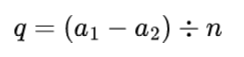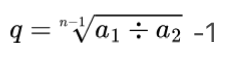• Preference Settings
From- To time of the change range
Theme
Bright
Classic black
Pitch black
• Exchange Rate Switch
Language
• 简体中文
• English
• Tiếng Việt
• 繁体中文
• Español
• Русский язык
• Français
• Deutsch
• Italiano
• Português
• ภาษาไทย
• Indonesia
• Türkçe
• 日本語
Exchange Rate Switch
• CNY
• USD
• KRW
• VND
• EUR
• GBP
• HKD
• JPY
• RUB
• TRY
• INR

### Gate.io Help Center

Customer Support / TicketGate.io > Help Center > Smart Quant > Introduction to Quantitative Strategy
##### More article in the group
Grid Interval and Grid Returns in Grid Trading##### Gate.io
Updated at:90 days 13 hours ago
lv

## To begin with, let’s take a closer look at the following formulas

Suppose the value of the common difference is q, the upper limit price is a1, the lower limit price is a2,and the number of grids is n.

#### Then the calculation formula for arithmetic grid interval q is:#### The calculation formula for geometric grid interval q is:### Returns Per Grid formula

Returns Per Grid = Common difference per grid * Purchase amount per grid * Number of the filled sell orders

## Grid Interval Formu

#### Take arithmetic grid interval as an example:Assuming that we are going to trade GT_USDT, the lower limit price of the grid is 30 USDT, the upper limit price is 150 USDT, and the grid quantity is 3, then the grid interval q=(150 - 30)/3 = 40. So the order price would be 30 USDT/70 USDT/110 USDT/150 USDT. Four positions in total.

A larger grid interval will cause a higher grid density and more frequent trades, but lower returns per grid; whereas a smaller interval will produce a lower grid density and lower trade frequency, but higher returns per grid.

## Grid Returns

Returns Per Grid = Common difference per grid * Purchase amount per grid * Number of the filled sell orders.

In this formula, the common difference per grid varies according to several major parameters, including "Price Range", "Number of Grids", and "Entry Price".

Common difference per grid = Selling Price(current order) - Buying Price(last order)

Essentially, the very first time when the bot executes the sell order of a grid, it will be matched with the entry price, following the second time of the sell order of the grid, which will be matched with the buying price of the last order. Therefore, the profit gaps between the grids could be as large as dozens of times.

For example, for the trading pair GT_USDT, the entry price is 30 USDT, the grid interval is 10 USDT, and the purchase amount per grid is 1. If the price for GT_USDT rises unilaterally to 280 USDT with no fluctuations, then the selling price per grid is 30 USDT, 40 USDT, 50 USDT... 280 USDT.

When the price for GT_USDT first hits 250 USDT, the grid will be sold at the entry price of 30 USDT. Then the common difference for this grid is 250 USDT - 30USDT = 220 USDT;

Immediately after the price for GT_USDT bounces back to 240 USDT, and rises up to 250 USDT again, the grid will be sold based on the last selling price of 240 USDT instead of the entry price of 30 USDT. So the common difference for this grid is 250 USDT - 240 USDT = 10 USDT;

Later, the price for GT_USDT goes up from 250 USDT to 280 USDT, the selling price of the grid when it first reached 280 USDT will automatically be matched with the entry price of 30USDT. At this time, the common difference for this grid is 280 USDT - 30 USDT = 250 USDT.

We can see from the above examples that common differences for the three grids are 220 USDT, 10 USDT, and 250 USDT respectively. There is even a 25-times spread between the lowest and the highest common difference, which is completely normal.

Can't find the answer you want?Submit a Ticket

Popular Articles

Articles